### Matched filter example

In the previous two examples we were concerned with frequency filters to separate the wanted signal from an unwanted signal.  This is a very frequent usage of filters.  However, in as much as there is noise in the frequency band of the signal, a frequency filter cannot really help to separate the noise from the useful signal.  If we need to do so, we will need to have more knowledge of the signal than just its frequency band (and hence, the information content of the signal will be less than the potential entropy of the signal in that band).  An extreme case of knowledge of the signal is the case where we know the entire shape of the signal: we only don't know when it will arrive and how large it will be.  These are the only two elements of information that we ignore about this signal (and hence, it are the only two sources of entropy of the signal).

We take an artificial example where an ultrasound pulse in the few 10 KHz range, with a very peculiar structure, is sent out, reflected and received.  The echo is so weak as to be totally swamped by noise.

The signal is, again, artificially constructed with a mathematical formula:

```sb = cos(180.02*t).*sin(8.81*sin(t)+2*t)+sin(168.3*t).*cos(9.21*sin(1.4*t)+2.1*t);
s = sb.*exp(-(t-tshift).^2/(2*twidth.^2));```

This is essentially a complicated modulated signal with a Gaussian envelope at a certain moment in time.  It looks like this: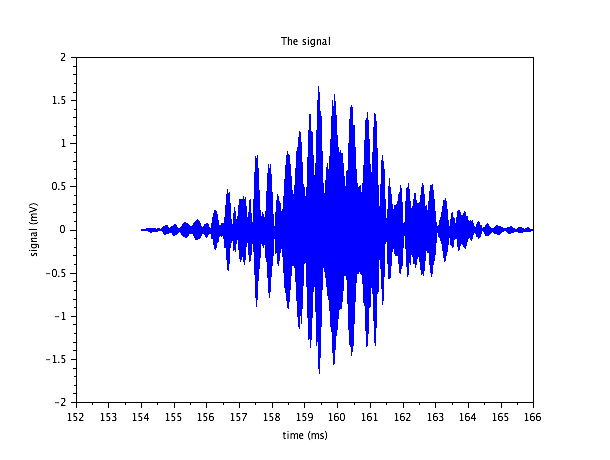As we can see, this is quite an involved signal structure.  The illustration doesn't even illustrate the individual oscillations which we only see if we zoom on a small part of it: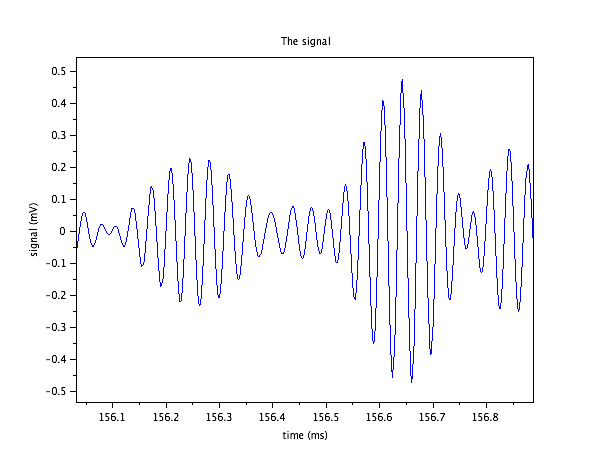If we now know that this pulse is the echo and will be part of the received signal, which will contain a lot of noise, the information we're after is essentially when this pulse will arrive (and eventually, how big it will be).  But let us suppose that this tiny echo is swamped by receiver noise which is much larger than the original signal.  We consider that the noise is flat (white noise) up to 250 KHz, with an RMS of 3 mV.  Note that the RMS value of the noise is larger than the maximum excursion of the signal pulse, so the signal will be totally invisible in the noise.

However, because our signal is much more narrow band than the noise, we may think that we can do as in the first example, where the signal also seemed swamped in the noise when looking at it, but where the narrow spectral band of the signal clearly stood out from the broad noise spectrum.  Let us look at the spectral densities of signal and noise: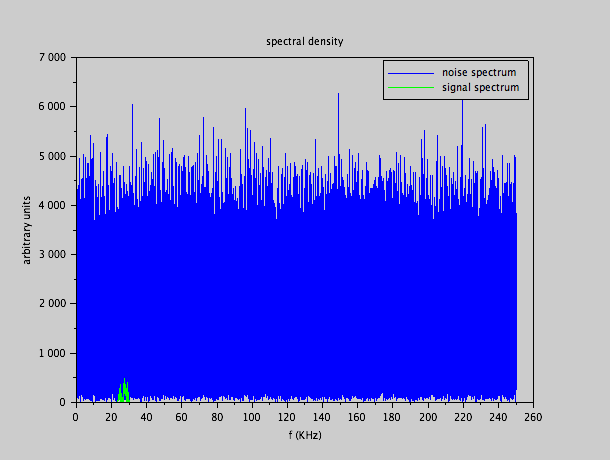We see that this time, also in the frequency domain, the signal is totally swamped by the noise.  However, this can still come about because our signal is much more concentrated in time (only a few milliseconds).  So we consider a perfect frequency filter that has a pass band from 23 KHz to 31 KHz, to cut out the useful band of the signal, and apply it to the noisy signal.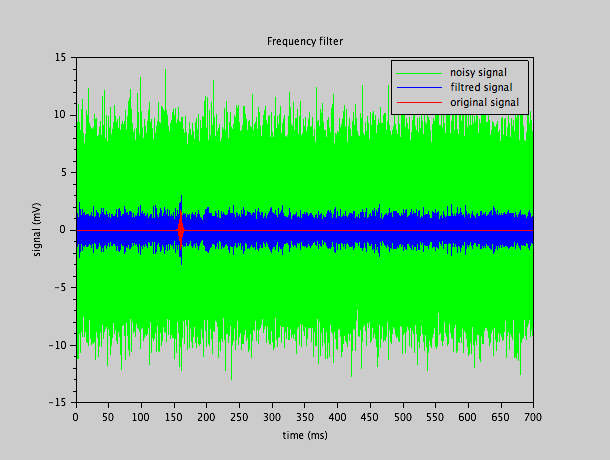The red signal is the pulse we're after, and the green signal is the raw input signal (which indeed swamps totally the red signal).  The blue signal is the output of the perfect frequency filter.  As we see, the frequency filter has seriously diminished the noise level, as it had done in example 1.   But we see that even the filtered signal has a noise level that is of the same amplitude as the wanted signal.  The wanted signal still doesn't stand out from the noise, although it is less swamped.  In other words, the sole knowledge of the useful frequency band of the signal is not sufficient to distinguish it clearly from the noise.

What we've done here, is in fact what we did in the first filter example, except that the noise contamination in the useful band of the signal is still too high.

We now apply (numerically, with a sample frequency of 500 KHz) a matched filter to the noisy signal.  The matched filter is matched to the signal pulse we described earlier, with a flat (white) noise spectrum.  In that case, the impulse response of the filter is the inverted signal pulse.  When this filter is applied to the signal, one obtains the following result as an output signal of the filter: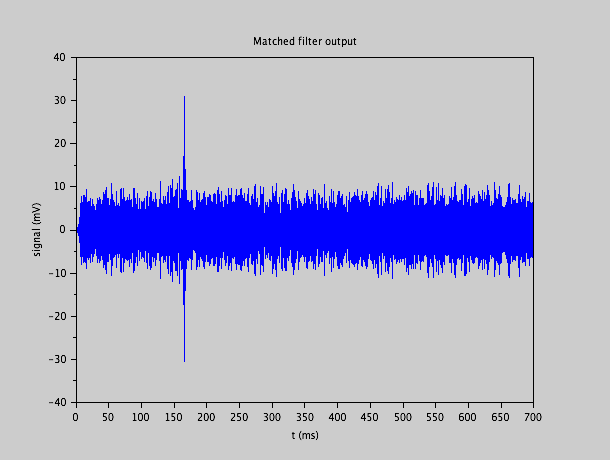We clearly see the 'glitch' around 165 ms, which indicates the presence of the signal at that moment.  The height of the glitch will signal the strength of the signal. Note that the matched filter doesn't reproduce a 'cleaned-up' version of the wanted signal ; it just produces a stronger output amplitude when the searched-for signal is detected, but the shape of the filter output signal doesn't look like the input signal.  This is not necessary, as, by definition of the matched filter, we know this shape.

When we zoom in on the filter output around the glitch, it takes on this form: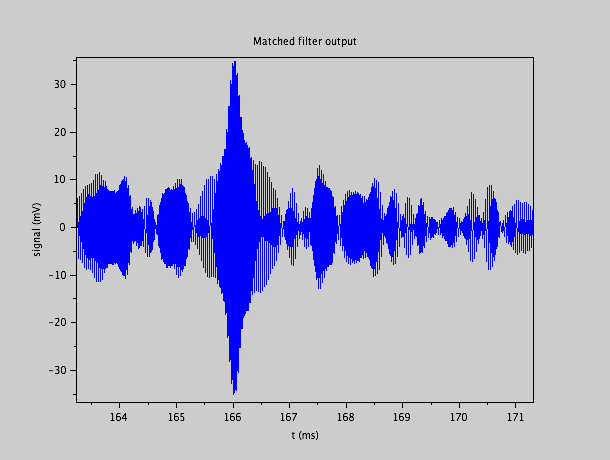from which we can deduce that the signal occurred - up to a fixed and known delay - at moment 166 ms.  If we zoom in even more, we see the individual oscillations at the output of the filter: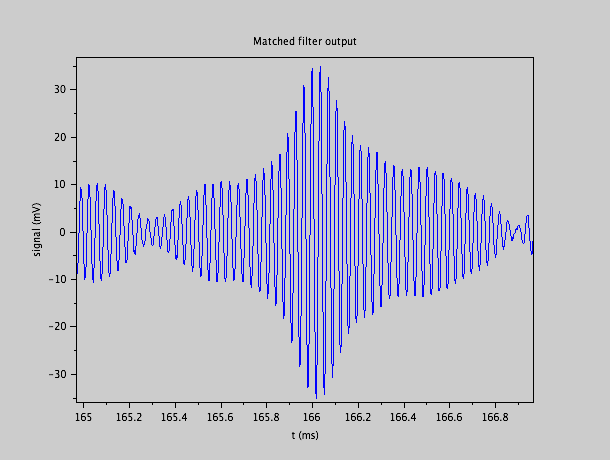We see that the time determination must be one of the peaks between 165.8 ms and 166.1 ms grossly.  It takes a more involved estimation if we want to fix confidence intervals.

In order to see the difference between the original signal, and the expected result of the matched filter, and also to determine the fixed delay between the actual signal and the peak output of the matched filter, it is sufficient to pass the pure signal (without noise) to the matched filter.  We obtain the following result: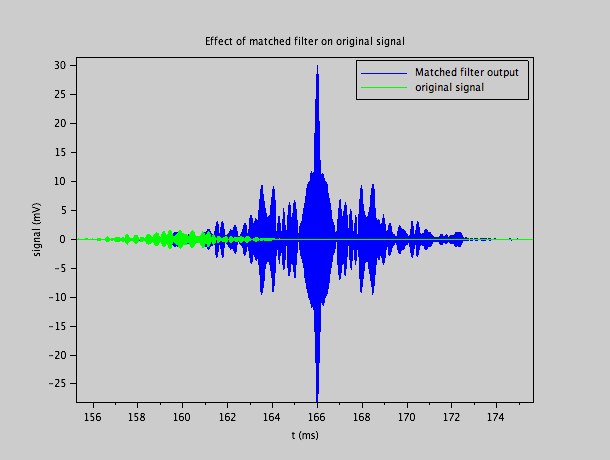We see that the peak value of the output occurs at a certain moment after the original (green) signal.  As the green signal is spread out in time, we have to define a certain "top" of this signal, and determine the delay between this top and the peak of the matched filter output.

We also note that the overall shape of what comes out of the matched filter doesn't look like the expected input signal.  The matched filter is just supposed to give as clear and as narrow an amplitude peak in the output when the signal is detected.

### Realisation

The only practical way to implement a matched filter with an involved signal shape, is a digital FIR filter with many taps.  Such a filter takes quite some computational resources.  Our example takes 6000 samples of the signal, which means that our filter will have 6000 FIR taps.  That is a lot.  It means that one has to apply 6000 multiplications, and 6000 additions per sample.  At a sample rate of 500 Ks/s, one arrives at 6 Gflops / s.   This can be achieved in high-end DSP cores, or in median FPGA solutions.  My personal preference would be an FPGA solution.

Of course, in this particular situation it is not necessary to have a digital bandwidth of 250 KHz, given that the useful signal is in the 30 KHz range, we could easily do with a 50 KHz bandwidth, or a 100 Ks/s rate.  This would bring the number of taps down by a factor of 5, so only 1200 FIR taps, and it would bring the computation rate down to 2400 FLOPs per sample (1200 multiplications, and 1200 additions), or 240 Mflops / s.  This is already much more reasonable, and any DSP can easily do that.  Even a relatively small FPGA can do so.  We would need, however, an analogue low pass anti-alias filter, cutting between 30 KHz and 50 KHz.  A 4-th order active Butterworth filter with a simple topology is probably a good choice.

As we see, a matched filter is capable of detecting the presence of a signal that is totally buried in noise.  The brute signal maximum amplitude is about a factor 6 or 7 smaller than the maximal noise excursions.  But even when looking at the narrow frequency band of the signal, we see that the signal level is of the same amplitude as the noise.  Up to now, we considered this a nuisance: we had a weak signal buried so much in noise that we had difficulties recovering it.   We typically considered the application of radar/sonar/ultrasound positioning, where the echo of the object under study is so small as to be below the noise level of the receiver.   We could increase the signal level by increasing the power of the emitter, and we must have some reason not to do so.  This can be a reason of power consumption, of cost, or another limitation of the output power of the emitter (for instance, we don't want the nearby sound signal to be so intense as to cause damage).  In this option, if the signal could be larger than the noise so that we don't need any matched filter, we would welcome that.

But having a signal below the noise can have the great advantage of being hardly detectable by others.  If one would like to transmit a message without being detected, then staying below the noise level of the channel used can be a great way not to attract any attention to the signal.  Of course this is hard to achieve in open air emissions from a localized source, because near the source the signal strength will often be over the ambient noise level.   If one spreads out the source over a certain area and if one uses a large spectral width, it is nevertheless possible to remain close to the ambient noise level and escape detection.   Without a matched filter, it is then impossible to recognize the signal, and in order to build a matched filter, one needs to know the shape of the transmitted signal.  Without that knowledge, it is then difficult or impossible to detect the fact that a signal is being sent, let alone that one can read the message.  We are in fact in a cryptographic situation, where the shape of the signal is the secret key.

Instead of using a complicated mathematical waveform, one can just as well take a sequence of 'white noise' as the signal.  As such, the signal is even statistically indistinguishable from genuine noise if it could be detected.  Only a matched filter with that particular sequence will detect the signal.

Let us look at this example which is similar to the previous one, except that we replaced the mathematical signal formula by white noise, included in the same Gaussian envelope: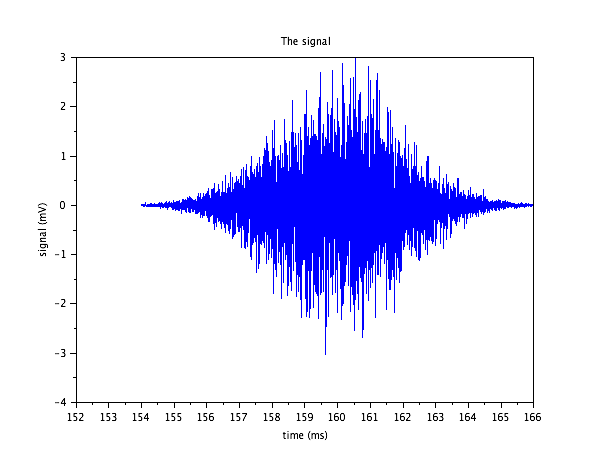The spectral density of this noise is quite similar to the white genuine noise that is added to it.  Only, because it is much more limited in time, and because it has a smaller amplitude, it is much much smaller: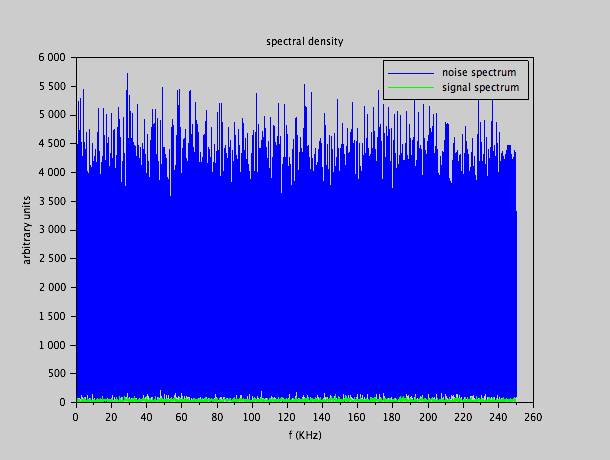As such, it seems impossible to detect.  As the bandwidth of the 'signal' is just as broad as the bandwidth of the genuine noise, of course no single frequency filter will make any distinction between the genuine noise and the signal.

However, a matched filter adapted to the exact noisy sequence will find it without the slightest difficulty.  The implementation is identical to the earlier example, only the coefficients at the 6000 taps change: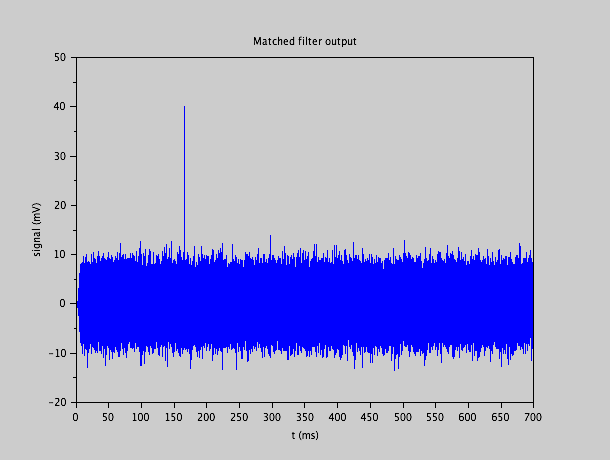The 'glitch' clearly stands out a factor of about 4 above the highest noise excursions and is very narrow in time.  Such a signal is essentially undetectable without the precise knowledge of its 'noise' sequence, which acts as secret key.

### Conclusion

The matched filter is capable of detecting the presence of a signal which is deeply buried under the noise level of the available signal at hand.  The price to pay is that one has to know the exact shape of the incoming signal, which limits the information that signal can carry to the moment in time and eventually, the amplitude.

In practice, the matched filter to an involved signal has to be implemented by a digital FIR filter, which implies quite some computation, even at relatively modest sampling frequencies.  The matched filter can also be used in stealth applications, where the signal is kept below the ambient noise level to avoid detection.